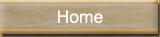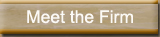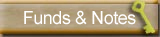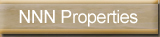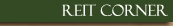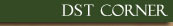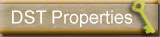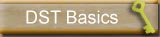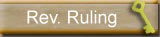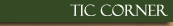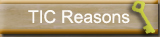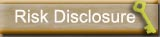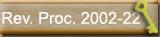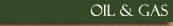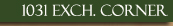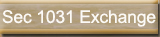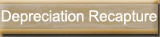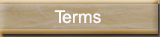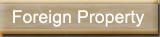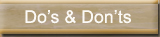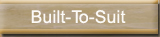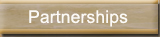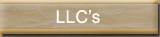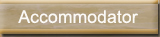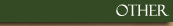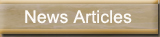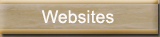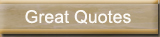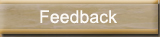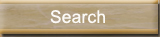# Depreciation Recapture

Capital gain tax rates are currently at historical lows but tax rules require investors to recapture at a higher tax rate (typically 25%) the portion of the gain on the sale that relates to allowable depreciation over the period the asset was held .  Therefore, depreciation recapture is a significant factor in motivating an investor to participate in a like-kind exchange.

The following discussion on the tax consequences of the depreciation recapture rules assumes that (1) your regular marginal income tax bracket is greater than 10% or 15%, and (2) the real estate sold is the only business asset sold by you in the tax year of the sale.

Generally, the gain from the sale by a noncorporate taxpayer of real estate that is a capital asset (or is used in a business) and is held more than 12 months isn't taxed at a rate higher than 15%.

But a more complex set of rules comes into play when the asset sold is depreciable real estate. This is so because, in that case, a maximum rate of 25% will apply to what's called unrecaptured section 1250 gain and a maximum rate of 15% will apply to the balance of the gain. �Unrecaptured section 1250 gain� refers to the portion of gain that is eligible for capital gain treatment even though it is attributable to previously allowable depreciation. A further complication is that the portion of the gain that is unrecaptured section 1250 gain depends, as shown below, on when the property was placed in service.

Property placed in service after 1986. For real estate placed in service after 1986, all depreciation deductions allowable before the sale of the real estate give rise to unrecaptured section 1250 gain. Thus, if you sell, at a gain of \$200,000, a building on which \$90,000 of depreciation deductions were allowable to you through the time of sale, \$90,000 of the gain is unrecaptured section 1250 gain that will be taxed at a rate of 25%. The remaining \$110,000 of the gain will be taxed at a rate of 15%.

Property placed in service before 1987 and after 1980. For real estate placed in service before 1987, but after 1980 (pre-1987 realty), the treatment of gain on sale depends on whether the real estate is residential or nonresidential.

Residential real estate. If you depreciated residential pre-1987 realty using just straight line depreciation, the tax results if you sell it will be the same as for a sale of post-1986 property, as described above. But if (as was possible) you, at any time, used a declining balance method to depreciate the real estate, the gain on sale would be taxed as follows:

• gain, to the extent of the depreciation claimed that exceeds what would have been allowable under straight-line depreciation, will be recaptured as ordinary income, and, thus, taxed at rates as high as 35% in 2003 and later years (�ordinary income rates�) (but the amount of excess depreciation subject to recapture may be less for certain low-income housing).
• gain, to the extent of the depreciation that isn't recaptured as ordinary income, will be taxed at a rate of 25%.
• the balance of the gain will be taxed at a rate of 15%.

Example. In January 1986, you paid \$1.3 million for an apartment building (not a low-income building), of which \$1 million was allocated to the improvements. You depreciated the property using the 175% declining balance method. You sold the property in July 2003 for \$2 million. From 1986 through 2003, a total of \$915,750 in depreciation was claimed. Assuming the only adjustment to basis was for depreciation, there would be a gain of \$1,615,750 (\$2 million less remaining basis of \$384,250), taxed as follows:

(a) \$19,583 (the excess of \$915,750 depreciation claimed over \$896,167 that would have been allowable using straight-line depreciation) would be taxed as ordinary income;

(b) \$896,167 (the depreciation that isn't recaptured as ordinary income under (a)) would be taxed at a rate of 25%;

(c) \$700,000 (total gain less amounts in (a) and (b)) would be taxed at a rate of 15%.

Nonresidential real estate. As is the case for residential pre-1987 realty, if you depreciated nonresidential pre-1987 realty using just straight-line depreciation, the tax results if you sell it will be the same as for a sale of post-1986 property, as described above. But if, as was possible, you, at any time, used a declining balance method to depreciate the realty, the gain on sale would be taxed as follows:

• gain, to the extent of the full amount of depreciation allowable to the time of sale, would be recaptured as ordinary income, and, thus, taxed at ordinary income rates;
• the balance of the gain would be taxed at a rate of 15%.

Example. Assume the same facts as in the Example above, except that the \$1.3 million building is a commercial building. The gain is the same, \$1,615,750, but would be taxed as follows:

(a) \$915,750 (representing all of the depreciation allowable) would be taxed as ordinary income;

(b) \$700,000 (the balance of the gain) would be taxed at a rate of 15%.

Pre-1981 property. The following rules apply if you sell real estate placed in service before 1981:

• the excess of depreciation claimed over straight-line depreciation is recaptured as ordinary income, and, thus, taxed at ordinary income rates (but the amount of excess depreciation subject to recapture may be less for certain residential real estate or for real estate acquired before 1970).
• gain, to the extent of the balance of depreciation allowable, is unrecaptured section 1250 gain, taxed at a rate of 25%.
• the balance of the gain, if any, would be taxed at a rate of 15%.

If you have further questions about the above rules or would like us to compute the potential tax that you face, please let us know.